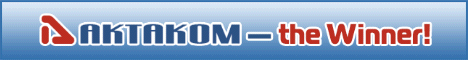Search

# HP-9150 Oscilloscope Probe 150 MHz Selectable X1/Ref/X10

 The HP-9150 is a passive high impedance oscilloscope probe designed and calibrated for use with instruments having an input impedance of 1MΩ. Switch Selectable x1/ x10/ Ref Probe.

The HP-9150 is a passive high impedance oscilloscope probe designed and calibrated for use with instruments having an input impedance of 1MΩ.

The probe incorporates a two position slide switch in the head which selects attenuation of x1 and x10.

X1 position:

• Input Impedance: R= 1Mohm, C= 68pf
• Bandwidth= 6MHz
• Max Voltage In(Vin)= 600V

X10 position:

• Input Impedance: R=10Mohm, C=18pf
• Bandwidth= 150MHz
• Max Voltage In(Vin)=600V

Back to the section